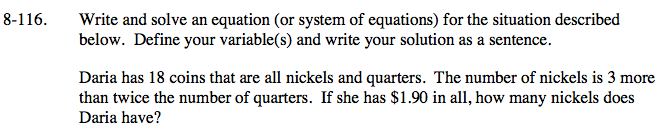### Home > CAAC > Chapter 8 > Lesson 8.3.3 > Problem8-116

8-116.n = # of nickels
q = # of quarters

Use the information in the problem to set up a system of equations.

2q + 3 = n

0.05n + 0.25q = 1.9

Substitute 2q + 3 in for n.

0.05(2q + 3) + 0.25q = 1.9

Solve for q.

0.1q + 0.15 + 0.25q = 1.9

0.35q = 1.75

q = 5

Substitute 5 into one of the original equations for q.

2(5) + 3 = n

10 + 3 = n

13 = n